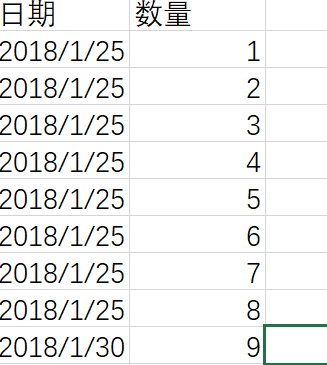python，读取csv文件，并画图，日期为横轴2个回答

https://blog.csdn.net/belldeep/article/details/78170274weixin_42793644 如何更改时间间隔和y值的间隔，谢谢啦Python+OpenCV计算机视觉

Python+OpenCV计算机视觉

python 画图，用csv,怎么修改时间间隔和x轴间隔

fig.autofmt_xdate() # 设置x轴时间外观 ax1 = fig.add_subplot(1,1,1) autodate = mdate.AutoDateLocator() ax1.xaxis.set_major_locator(autodate) # 设置时间间隔 # 设置时间标签显示格式 dateFmt = mdate.DateFormatter('%y%m%d') ax1.xaxis.set_major_formatter(dateFmt) # 将x轴次刻度标签设置为61的倍数 xminorLocator = MultipleLocator(61) # 显示次刻度标签的位置,没有标签文本 ax1.xaxis.set_minor_locator(xminorLocator) #ax1.set_xticks() # 设置间隔 ax1.set_xlim(date2num('2018/01/02'),date2num('2018/01/29')) # 设置x轴范围 plt.xticks(rotation=90) # 显示日期旋转90度 plt.title(u'图') plt.plot(x,y, label='') plt.grid(True) plt.xlabel(u'日期') plt.ylabel(u'百分比') plt.legend(loc=2,prop={'family':'SimHei','size':12}) # loc=2 : upper left plt.savefig("图.png", dpi=100) plt.show()

python中的画图坐标问题

python的pandas模块画图时中文显示不完全

python 画图plot画图时数据太多，图看起来不好看。请问怎么拉长X轴，或者增加每个点之间的间隔

python 用matplotlib绘图，如何让多个子图的横轴标签全部旋转

python画图时，怎么设置坐标轴的单位长度？

echarts 横轴时间显示有问题

matplotlib画图时坐标轴范围和刻度无法正常设置，未报错

import csv import warnings warnings.simplefilter(action='ignore', category=FutureWarning) from datetime import datetime from matplotlib import pyplot as plt file_path=r'C:\Users\YSRD-B-0281\Desktop\forth.csv' with open(file_path) as f: reader=csv.reader(f) header_row=next(reader) dates,ohs=[],[] for row in reader: current_date=datetime.strptime(row,"%Y/%m/%d %H:%M") dates.append(current_date) oh=float(row) ohs.append(row) fig=plt.figure(dpi=128,figsize=(10,6)) plt.plot(dates,ohs,c='brown',alpha=0.5) plt.title("E4",fontsize=24) plt.ylim((0,120)) plt.yticks([0,20,40,60,80,100]) plt.show() plt.close()![图片说明](https://img-ask.csdn.net/upload/201907/25/1564039180_988567.png)

R语言时间序列画图横坐标问题

extjs实现曲线图，以时间为横轴

matplotlib画pie饼图的时候，有几个块的label说明是重叠起来的

2019 Python开发者日-培训

2019 Python开发者日-培训

150讲轻松搞定Python网络爬虫

150讲轻松搞定Python网络爬虫

YOLOv3目标检测实战：训练自己的数据集

YOLOv3目标检测实战：训练自己的数据集

java后台+微信小程序 实现完整的点餐系统

java后台+微信小程序 实现完整的点餐系统

2019 AI开发者大会

2019 AI开发者大会

4小时玩转微信小程序——基础入门与微信支付实战

4小时玩转微信小程序——基础入门与微信支付实战

Git 实用技巧

Git 实用技巧

Python数据清洗实战入门

Python数据清洗实战入门

MySQL数据库从入门到实战应用

MySQL数据库从入门到实战应用

.net core快速开发框架

.net core快速开发框架

Python数据挖掘简易入门

Python数据挖掘简易入门

Windows版YOLOv4目标检测实战：训练自己的数据集

Windows版YOLOv4目标检测实战：训练自己的数据集

HoloLens2开发入门教程

HoloLens2开发入门教程

Java8零基础入门视频教程

Java8零基础入门视频教程